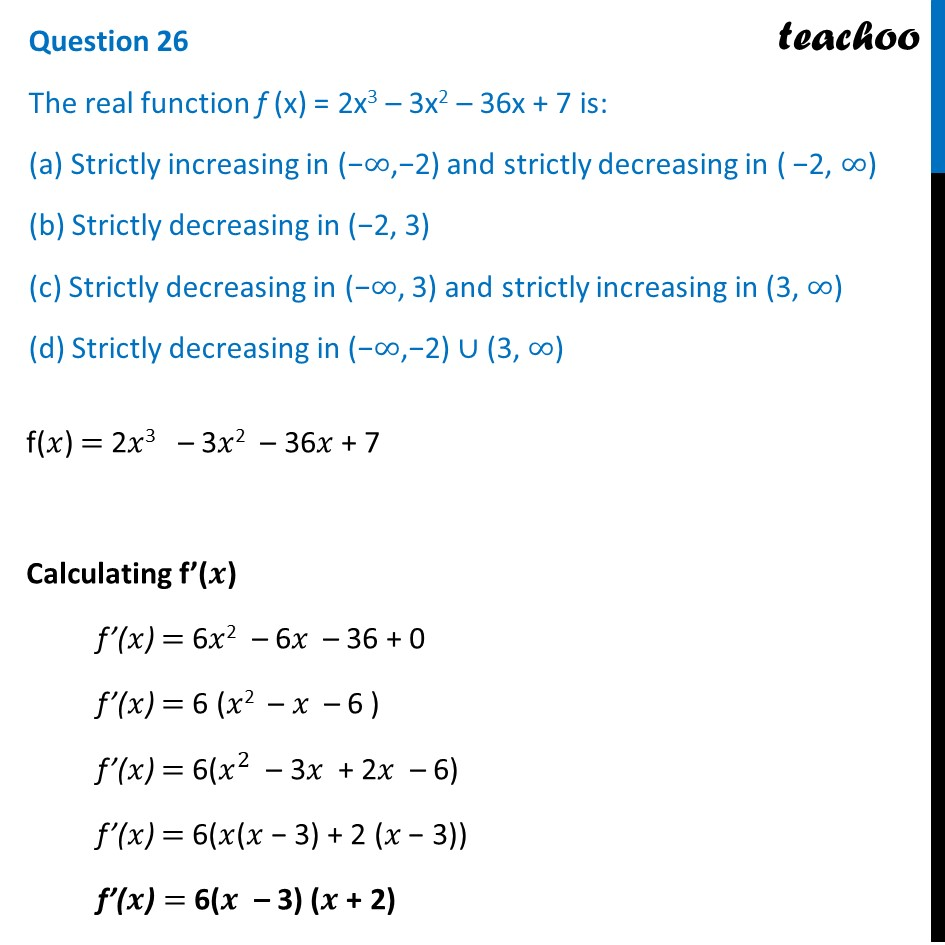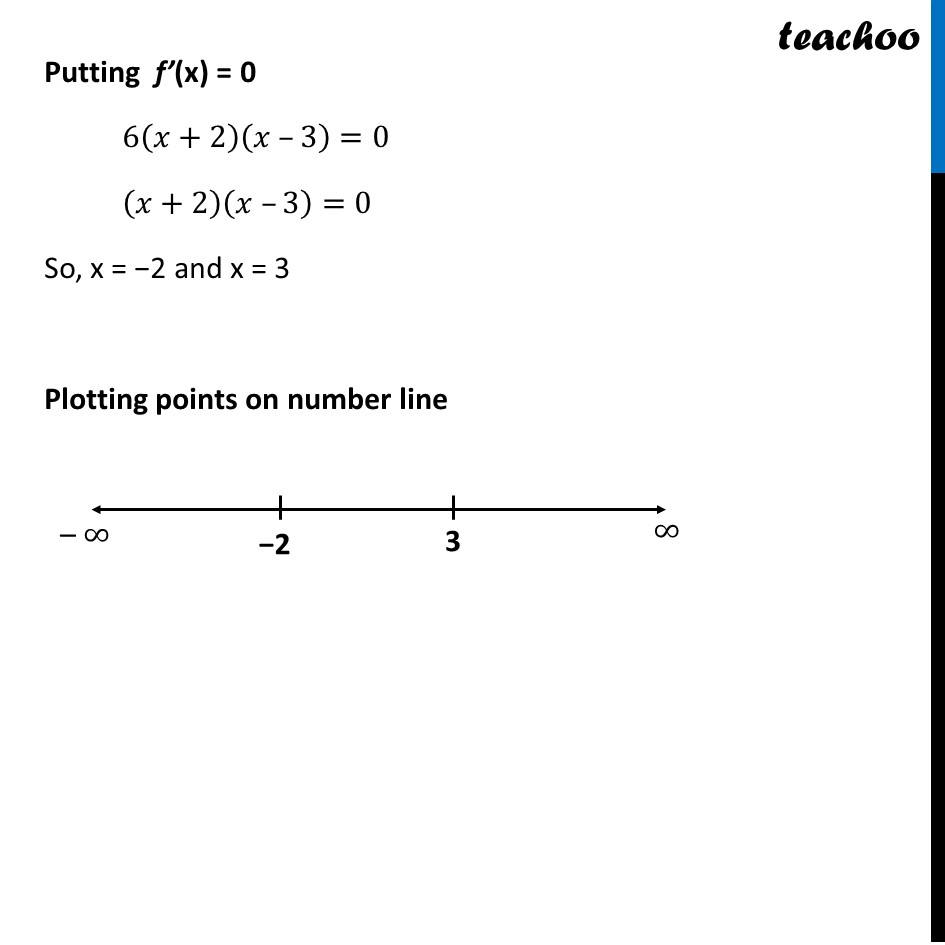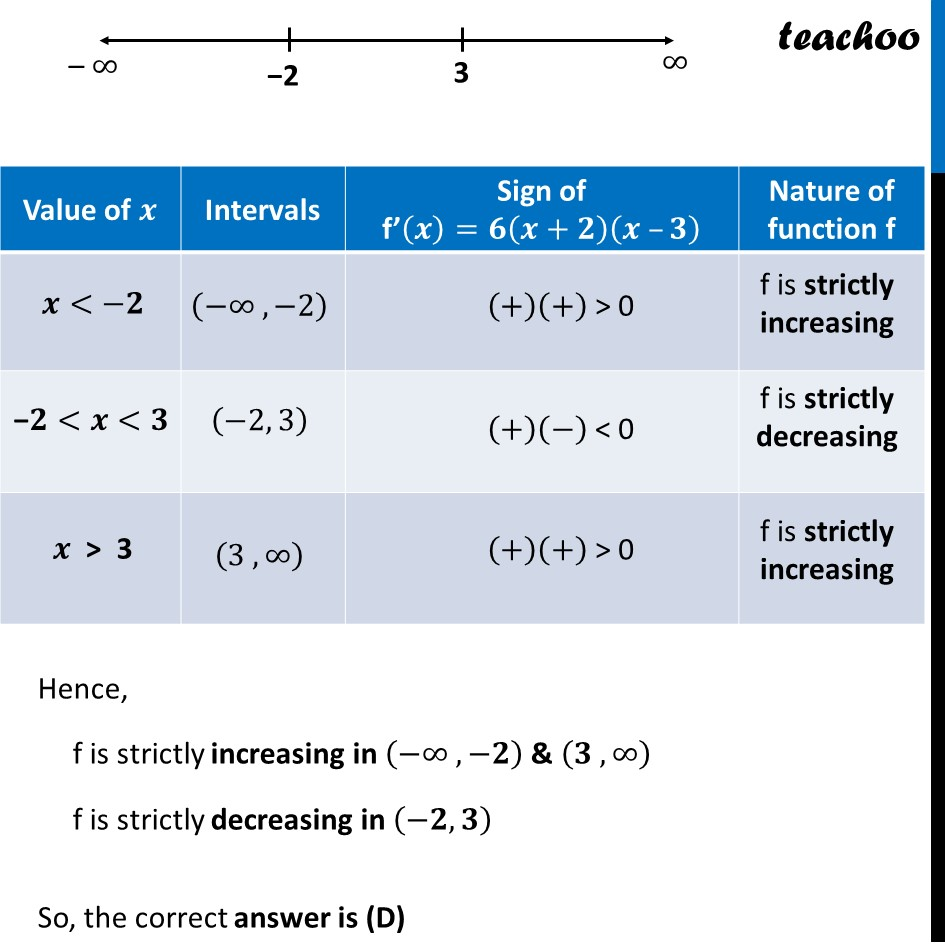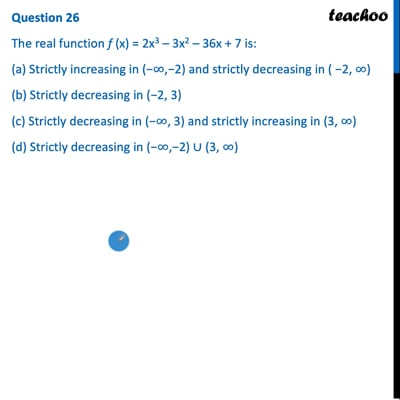CBSE Class 12 Sample Paper for 2022 Boards (MCQ Based - for Term 1)

Class 12
Solutions of Sample Papers and Past Year Papers - for Class 12 Boards

## (a) Strictly increasing in (−∞,−2) and strictly decreasing in ( −2, ∞) (b) Strictly decreasing in (−2, 3) (c) Strictly decreasing in (−∞, 3) and strictly increasing in (3, ∞) (d) Strictly decreasing in (−∞,−2) ∪ (3, ∞)

This question is inspired from Ex 6.2, 5 - Chapter 6 Class 12 - Application of DerivativesThis video is only available for Teachoo black users

Learn in your speed, with individual attention - Teachoo Maths 1-on-1 Class

### Transcript

Question 26 The real function f (x) = 2x3 – 3x2 – 36x + 7 is: (a) Strictly increasing in (−∞,−2) and strictly decreasing in ( −2, ∞) (b) Strictly decreasing in (−2, 3) (c) Strictly decreasing in (−∞, 3) and strictly increasing in (3, ∞) (d) Strictly decreasing in (−∞,−2) ∪ (3, ∞) f(𝑥) = 2𝑥3 – 3𝑥2 – 36𝑥 + 7 Calculating f’(𝒙) f’(𝑥) = 6𝑥2 – 6𝑥 – 36 + 0 f’(𝑥) = 6 (𝑥2 – 𝑥 – 6 ) f’(𝑥) = 6(𝑥^2 – 3𝑥 + 2𝑥 – 6) f’(𝑥) = 6(𝑥(𝑥 − 3) + 2 (𝑥 − 3)) f’(𝒙) = 6(𝒙 – 3) (𝒙 + 2) Putting f’(x) = 0 6(𝑥+2)(𝑥 –3)=0 (𝑥+2)(𝑥 –3)=0 So, x = −2 and x = 3 Plotting points on number line Hence, f is strictly increasing in (−∞ ,−𝟐) & (𝟑 ,∞) f is strictly decreasing in (−𝟐, 𝟑) So, the correct answer is (D)